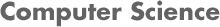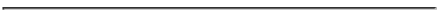### CS2223 Algorithms Homework 4 Solutions - D Term 2009

#### PROF. CAROLINA RUIZ• Homework Problems:

1. (40 Points) Problem 1. Suppose that your MP3 player has a disk capacity of M megabytes. Also assume that you have the following n songs available for download: S1, S2, S3, ..., Sn. The ith song Si takes up mi megabytes. The problem is that your MP3 player capacity, M, is less than the sum of all the mi's. That is, M < Σni=1 mi.
1. (20 Points) Assume that you want to maximize the number of songs that you download onto your MP3 player. Prove or give a counterexample: A greedy algorithm that selects songs to download in increasing size order (from smallest to largest) will produce an optimal solution.

Solution: Yes, this greedy strategy will produce an optimal solution. Here is the proof:

The algorithm starts by sorting the songs in increasing size order. Assume that the resulting sorting is: Sg1, Sg2, Sg3, ..., Sgn. Now, the algorithm will select the first k songs on this resulting list, Sg1, Sg2, Sg3, ..., Sgk, where k is such that Σki=1 mgi ≤ M, but Σk+1i=1 mgi > M.

Assume by way of contradiction, that the solution produced by this greedy algorithm (Sg1, Sg2, Sg3, ..., Sgk) is not optimal. Hence there must exist a different solution Sd1, Sd2, Sd3, ..., Sdq where q > k (that is, this solution contains more songs than the one produced by the greedy algorithm). Assume that the different solution is sorted in increasing size order: that is, md1 ≤ md2 ≤ md3 ≤ ... ≤ mdq.

Let's compare these two solutions position by position and let j+1 be the first position where the two sequences differ:  Sg1, Sg2, Sg3, ..., Sgj, Sg(j+1), Sg(j+2), ..., Sgk greedy solution Sd1, Sd2, Sd3, ..., Sdj, Sd(j+1), Sd(j+2), ..., Sdk, ..., Sdq different solution
The greedy algorithm selected Sg(j+1) because it was the smallest song not yet selected (i.e., smallest song not in Sg1,..., Sgj). The different solution selected a different song Sd(j+1) and hence it must hold that mg(j+1) ≤ md(j+1), and also that Σj+1i=1 mgi ≤ Σj+1i=1 mdi. So the greedy solution "stays ahead" of the different solution. We can continue this reasoning by induction and show that for each x, 1 ≤ x ≤ q, mgx ≤ mdx, and Σxi=1 mgi ≤ Σxi=1 mdi.

But now, the greedy algorithm stopped selecting songs after the k-th one. As stated above, this means that Σki=1 mgi ≤ M, but Σk+1i=1 mgi > M. Nevertheless, the different solution picked more songs. However, Σk+1i=1 mdi ≥ Σk+1i=1 mgi > M. Hence, the different solution is not even a correct solution as it picked songs that exceeded the disk space limit M. This is a contradiction with the assumption that the different solution was indeed a solution better than the greedy one, and hence the greedy solution is optimal in terms of the number of songs selected.

2. (20 Points) Assume that you want to maximize the disk utilization of your MP3 player (that is, you want to use as many of your M megabytes as possible). Prove or give a counterexample: A greedy algorithm that selects songs to download in decreasing size order (from largest to smallest) will produce an optimal solution.

Solution: This greedy strategy is not optimal. Consider the following counterexample. Let the sizes of the songs be 5, 4, 3, and 1.5 megabytes. Let the remaining disk space in your MP3 player be 10 megabytes. The greedy strategy would choose the songs with sizes 5 and 4. The disk utilization will be 9 megabytes (that is 90%). However, choosing the songs with sizes 5, 3, and 1.5 would be a better solution as it would use 9.5 megabytes (that is, 95%).

2. (160 points) Huffman's Optimal Prefix Codes Algorithm
Before you can solve this exercise, you need to read Sections 2.5 and 4.8 of the textbook in detail.

Solution:

Included below are the files containing Shweta Srivastava's Java implementation of heaps, priority queues, treeNodes, and Huffman's algorithm: